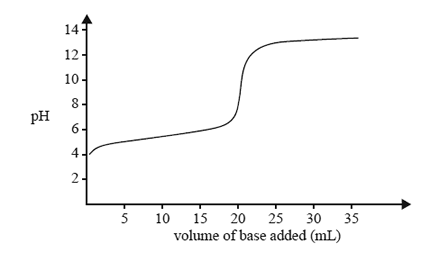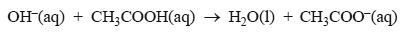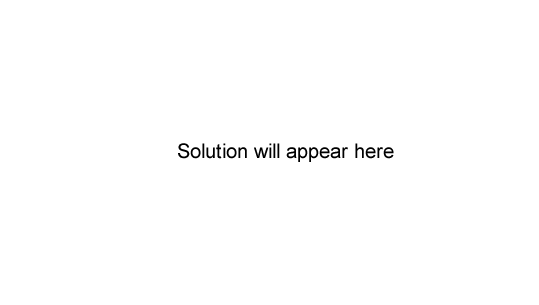Volumetric analysis (2013 VCE) 1) The change in pH as a 0.10 M solution of a strong base is added to 20.0 mL of a 0.10 M solution of a weak acid is shown below.Refer to the acid-base indicator data provided in the data book and identify the indicator that would be least suitable to detect the end point of this neutralisation. A. phenol red B. thymol blue C. phenolphthalein D. bromothymol blue Solution2) A 20.00 mL aliquot of 0.200 M CH3COOH (ethanoic acid) is titrated with 0.150 M NaOH. The equation for the reaction between the ethanoic acid and NaOH solution is represented asa) What volume of the NaOH solution is required to completely react with the ethanoic acid? Solutionb) Define the terms 'equivalence point' and 'end point'. Solutionc) Write an expression for the acidity constant of ethanoic acid. Solution The question below requires the student to have knowledge of chemical equilibria, a topic which comes up in unit 4 in 2014. d) Calculate the pH of the 0.200 M ethanoic acid solution before any NaOH solution has been added. Assume that the equilibrium concentration of the ethanoic acid is 0.200 M. Solutiond) Consider the point in the titration where the volume of NaOH added is exactly half that required for complete neutralisation. Select the statement that best describes the relative concentrations of ethanoic acid and ethanoate ions at this point. i) The concentration of ethanoic acid is less than the concentration of ethanoate ions. ii) The concentration of ethanoic acid is equal to the concentration of ethanoate ions. iii) The concentration of ethanoic acid is greater than the concentration of ethanoate ions. Solutione) What is the relationship between the concentration of H3O+and Ka at this point? Solution3) In a titration, a 25.00 mL titre of 1.00 M hydrochloric acid neutralised a 20.00 mL aliquot of sodium hydroxide solution. If, in repeating the titration, a student failed to rinse one of the pieces of glassware with the appropriate solution, the titre would be A. equal to 25.00 mL if water was left in the titration flask after final rinsing. B. less than 25.00 mL if the final rinsing of the burette is with water rather than the acid. C. greater than 25.00 mL if the final rinsing of the 20.00 mL pipette is with water rather than the base. D. greater than 25.00 mL if the titration flask had been rinsed with the acid prior to the addition of the aliquot. Solution4) In volumetric analysis, the properties of the reactants, as well as the nature of the reaction between them, will determine if a back titration is to be used. Consider the following cases. I The substance being analysed is volatile. II The substance being analysed is insoluble in water but is soluble in dilute acid. III The end point of the reaction is difficult to detect. In which cases would a back titration be more suitable than a simple forward titration? A. I and II only B. I and III only C. II and III only D. I, II and III Solution